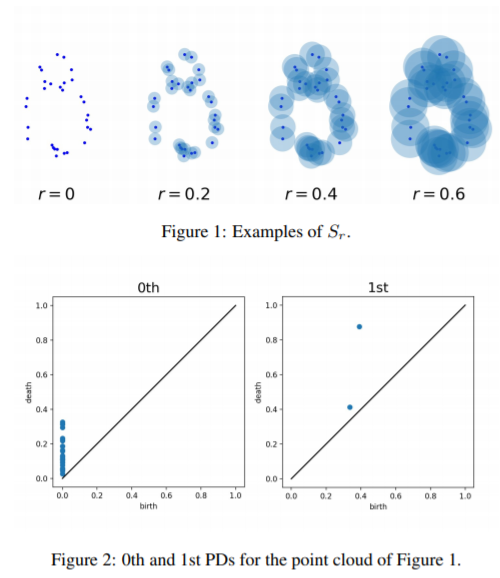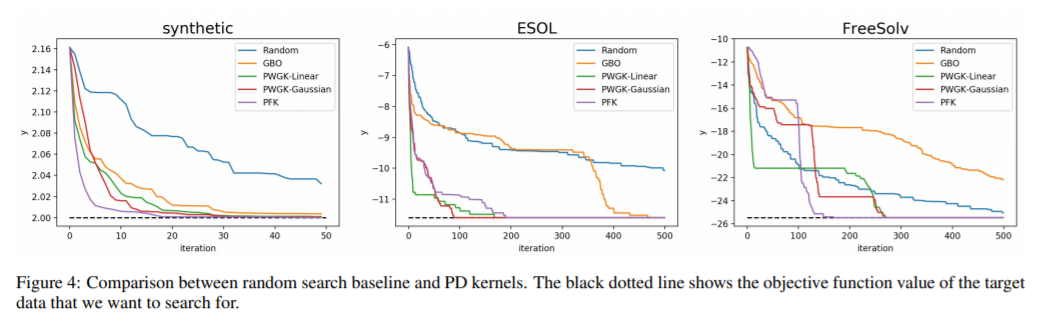### VIP内容0+1+0+

### 热门内容0+36+0+

### 最新内容The $\ell_0$-constrained empirical risk minimization ($\ell_0$-ERM) is a promising tool for high-dimensional statistical estimation. The existing analysis of $\ell_0$-ERM estimator is mostly on parameter estimation and support recovery consistency. From the perspective of statistical learning, another fundamental question is how well the $\ell_0$-ERM estimator would perform on unseen samples. The answer to this question is important for understanding the learnability of such a non-convex (and also NP-hard) M-estimator but still relatively under explored. In this paper, we investigate this problem and develop a generalization theory for $\ell_0$-ERM. We establish, in both white-box and black-box statistical regimes, a set of generalization gap and excess risk bounds for $\ell_0$-ERM to characterize its sparse prediction and optimization capability. Our theory mainly reveals three findings: 1) tighter generalization bounds can be attained by $\ell_0$-ERM than those of $\ell_2$-ERM if the risk function is (with high probability) restricted strongly convex; 2) tighter uniform generalization bounds can be established for $\ell_0$-ERM than the conventional dense ERM; and 3) sparsity level invariant bounds can be established by imposing additional strong-signal conditions to ensure the stability of $\ell_0$-ERM. In light of these results, we further provide generalization guarantees for the Iterative Hard Thresholding (IHT) algorithm which serves as one of the most popular greedy pursuit methods for approximately solving $\ell_0$-ERM. Numerical evidence is provided to confirm our theoretical predictions when implied to sparsity-constrained linear regression and logistic regression models.0+0+0+下载预览

### 最新论文The $\ell_0$-constrained empirical risk minimization ($\ell_0$-ERM) is a promising tool for high-dimensional statistical estimation. The existing analysis of $\ell_0$-ERM estimator is mostly on parameter estimation and support recovery consistency. From the perspective of statistical learning, another fundamental question is how well the $\ell_0$-ERM estimator would perform on unseen samples. The answer to this question is important for understanding the learnability of such a non-convex (and also NP-hard) M-estimator but still relatively under explored. In this paper, we investigate this problem and develop a generalization theory for $\ell_0$-ERM. We establish, in both white-box and black-box statistical regimes, a set of generalization gap and excess risk bounds for $\ell_0$-ERM to characterize its sparse prediction and optimization capability. Our theory mainly reveals three findings: 1) tighter generalization bounds can be attained by $\ell_0$-ERM than those of $\ell_2$-ERM if the risk function is (with high probability) restricted strongly convex; 2) tighter uniform generalization bounds can be established for $\ell_0$-ERM than the conventional dense ERM; and 3) sparsity level invariant bounds can be established by imposing additional strong-signal conditions to ensure the stability of $\ell_0$-ERM. In light of these results, we further provide generalization guarantees for the Iterative Hard Thresholding (IHT) algorithm which serves as one of the most popular greedy pursuit methods for approximately solving $\ell_0$-ERM. Numerical evidence is provided to confirm our theoretical predictions when implied to sparsity-constrained linear regression and logistic regression models.0+0+0+下载预览

Top